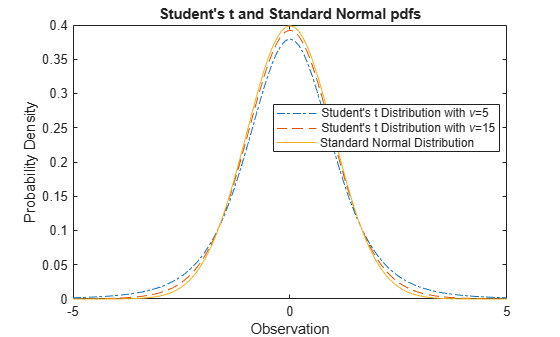Documentation

## Student's t Distribution

### Overview

The Student’s t distribution is a family of curves depending on a single parameter ν (the degrees of freedom).

### Parameters

The Student’s t distribution uses the following parameter.

ParameterDescription
ν = 1, 2, 3,...Degrees of freedom

### Probability Density Function

#### Definition

The probability density function (pdf) of the Student's t distribution is

$y=f\left(x|\nu \right)=\frac{\Gamma \left(\frac{\nu +1}{2}\right)}{\Gamma \left(\frac{\nu }{2}\right)}\frac{1}{\sqrt{\nu \pi }}\frac{1}{{\left(1+\frac{{x}^{2}}{\nu }\right)}^{\frac{\nu +1}{2}}}$

where ν is the degrees of freedom and Γ( · ) is the Gamma function. The result y is the probability of observing a particular value of x from a Student’s t distribution with ν degrees of freedom.

#### Plot

This plot shows how changing the value of the degrees of freedom parameter ν alters the shape of the pdf. Use tpdf to compute the pdf for values x equals 0 through 10, for three different values of ν. Then plot all three pdfs on the same figure for a visual comparison.

x = [0:.1:10]; y1 = tpdf(x,5); % For nu = 5 y2 = tpdf(x,25); % For nu = 25 y3 = tpdf(x,50); % For nu = 50 figure; plot(x,y1,'Color','black','LineStyle','-') hold on plot(x,y2,'Color','red','LineStyle','-.') plot(x,y3,'Color','blue','LineStyle','--') legend({'nu = 5','nu = 25','nu = 50'}) hold off#### Random Number Generation

Use trnd to generate random numbers from the Student’s t distribution. For example, the following generates a random number from a Student’s t distribution with degrees of freedom ν equal to 10.

nu = 10; r = trnd(nu)
r = 1.0585 

#### Relationship to Other Distributions

As the degrees of freedom ν goes to infinity, the t distribution approaches the standard normal distribution.

If x is a random sample of size n from a normal distribution with mean μ, then the statistic

$t=\frac{\overline{x}-\mu }{s/\sqrt{n}}$

where $\overline{x}$ is the sample mean and s is the sample standard deviation, has Student's t distribution with n – 1 degrees of freedom.

The Cauchy distribution is a Student’s t distribution with degrees of freedom ν equal to 1. The Cauchy distribution has an undefined mean and variance.

### Cumulative Distribution Function

#### Definition

The cumulative distribution function (cdf) of Student’s t distribution is

$p=F\left(x|\nu \right)={\int }_{-\infty }^{x}\frac{\Gamma \left(\frac{\nu +1}{2}\right)}{\Gamma \left(\frac{\nu }{2}\right)}\frac{1}{\sqrt{\nu \pi }}\frac{1}{{\left(1+\frac{{t}^{2}}{\nu }\right)}^{\frac{\nu +1}{2}}}dt$

where ν is the degrees of freedom and Γ( · ) is the Gamma function. The result p is the probability that a single observation from the t distribution with ν degrees of freedom will fall in the interval [–∞, x].

#### Plot

This plot shows how changing the value of the parameter ν alters the shape of the cdf. Use tcdf to compute the cdf for values x equals 0 through 10, for three different values of ν. Then plot all three cdfs on the same figure for a visual comparison.

x = [0:.1:10]; y1 = tcdf(x,5); % For nu = 5 y2 = tcdf(x,25); % For nu = 25 y3 = tcdf(x,50); % For nu = 50 figure; plot(x,y1,'Color','black','LineStyle','-') hold on plot(x,y2,'Color','red','LineStyle','-.') plot(x,y3,'Color','blue','LineStyle','--') legend({'nu = 5','nu = 25','nu = 50'}) hold off#### Inverse cdf

Use tinv to compute the inverse cdf of the Student’s t distribution.

p = .95; nu = 50; x = tinv(p,nu)
x = 1.6759 

### Mean and Variance

The mean of the Student’s t distribution is

$\text{mean}=0$

for degrees of freedom ν greater than 1. If ν equals 1, then the mean is undefined.

The variance of the Student’s t distribution is

$\mathrm{var}=\frac{\nu }{\nu -2}$

for degrees of freedom ν greater than 2. If ν is less than or equal to 2, then the variance is undefined.

Use tstat to compute the mean and variance of a Student’s t distribution. For example, the following computes the mean and variance of a Student’s t distribution with degrees of freedom ν equal to 10.

nu = 10; [m,v] = tstat(nu)
m = 0 
v = 1.2500 

### Example

#### Compare Student's t and Normal Distribution pdfs

The Student’s t distribution is a family of curves depending on a single parameter ν (the degrees of freedom). As the degrees of freedom ν goes to infinity, the t distribution approaches the standard normal distribution. Compute the pdfs for the Student's t distribution with the parameter nu = 5 and the Student's t distribution with the parameter nu = 25. Compute the pdf for a standard normal distribution.

x = -5:0.1:5; y1 = tpdf(x,5); y2 = tpdf(x,15); z = normpdf(x,0,1);

Plot the Student's t pdfs and the standard normal pdf on the same figure. The standard normal pdf has shorter tails than the Student's t pdfs.

plot(x,y1,'-.',x,y2,'--',x,z,'-') legend('Student''s t Distribution with \nu=5', ... 'Student''s t Distribution with \nu=25', ... 'Standard Normal Distribution','Location','best') title('Student''s t and Standard Normal pdfs')